# C++ 练气期之指针所指何处 - 一枚大果壳

Go to the source link to view the article. You can view the picture content, updated content and better typesetting reading experience. If the link is broken, please click the button below to view the snapshot at that time.

`指针`是一种`C++`数据类型，用来描述内存地址。

`内存`中的每一个存储单元格都有自己的地址，地址是使用二进制进行编码。`地址`从形态上看是一个整型数据类型。但是，它的数据含义并不表示数字，而是一个位置标志，类似于门牌号。• 在地址上`加上``减去`一个正整数，表示向前或向后移动地址。移动地址的意义：可实现从一个存储位置到达另一个存储位置。
• 地址与地址之间也可以相减，表示两个地址之间的差距。
• 地址与地址之间不可以相加、相乘、相除运算。对地址进行相加、相乘、相除类似门牌号门牌号之间相加、相乘、相除，没有任何意义可言。

## 2. 指针变量

`变量名`是变量的逻辑地址，由开发者提供。而变量地址是变量的物理地址，指变量在内存中的具体位置。如下声明语句，在编译时，编译器会做一些细碎的底层工作。

``````int num=20;
``````
• 根据数据类型的约定，在`内存`中找到一个可用的内存块。`int`一般大小为 `4B`
• 获取到内存块的物理地址，并把物理地址和开发者提供的变量名（逻辑名）进行关联，并保存在映射表中。
• 把数字 `20`保存在`num`变量中。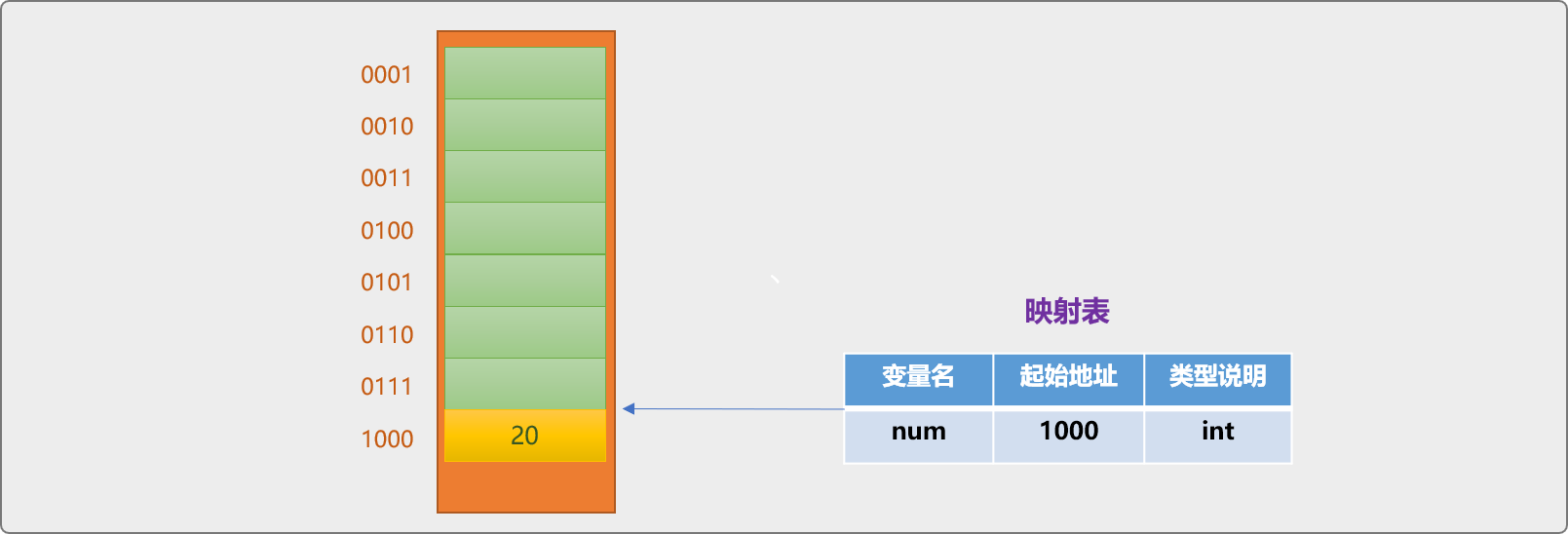``````std::cout<<num;
//输出结果：20
``````

`指针变量`也是内存中的一个存储块，只是变量中存储的是另一个`变量`在内存中的地址。如下代码，保存 `num`变量在内存的地址。

``````//整型类型变量
int num=20;
//指针类型的变量
int* num_p=&num;
``````

• `int *`表示`指针类型`。声明指针变量时，需要指定变量是用来保存`指针类型`数据。

`int *`表示指针变量是用来保存一个 `int`类型变量的地址，并不是指变量用来保存一个整型数据。

• `&`运算符，取地址运算符。`&num`表示获取`num`变量的内存（物理）地址。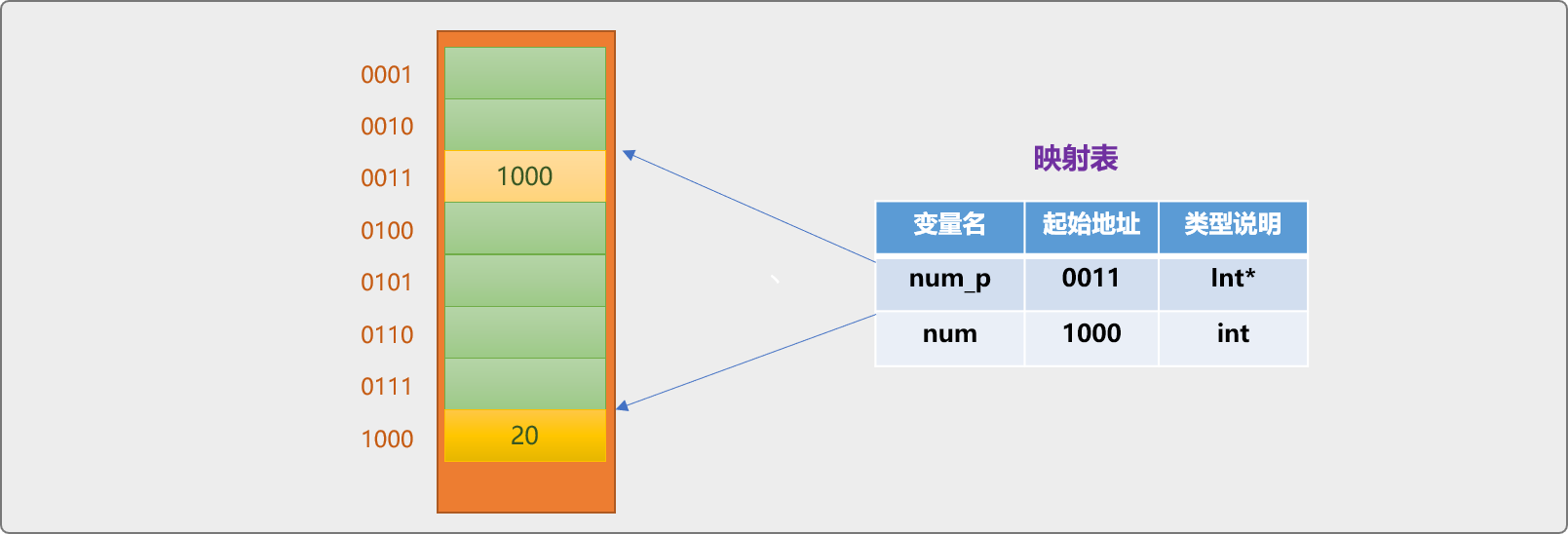``````std::cout<<num_p;
``````

``````std::cout<<*num_p;
//输出：20
``````

• 声明时`*`表示指针类型。`int* num_p;`
• 使用指针变量时，表示通过地址找到变量中的数据。

`*num_p``num`是访问同一个变量的两种方案。前者是使用物理名（内存地址）访问变量的语法，后者是使用逻辑名（变量名）访问变量。

``````int num=20;
int* num_p=&num;
//通过指针变量赋值，和 num=30 等同
*num_p=30;
std::cout<<*num_p<<std::endl;
std::cout<<num<<std::endl;
//输出结果：
30
30
``````

## 3. 几个问题

### 3.1 为什么要使用指针变量

• 访问速度。

``````int nums={1,2,3,4};
int* nums_p=nums;
for(int i=0;i<4;i++){
std::cout<<*(nums_p+i)<<std::endl;
}
//输出
1
2
3
4
``````

`数组变量`本质是指针变量，保存着数组在内存中的首地址。所以在把数组的地址赋值另一个指针变量时，`int* nums_p=nums;`是不需要使用`&`符号的。

• 访问 `new` 创建的内存块。

``````int *num01=new int;
``````

`new`运算符会在堆中开辟一个用来保存`int`类型数据的存储块，返回存储块的内存地址（指针类型数据） ，这时只能使用指针变量保存，并且通过指针变量使用这个存储块 。

``````int *num01=new int;
*num01=40;
std::cout<<*num01<<std::endl;
//输出：40
``````
• 使用指针变量作为函数的参数，用来影响函数调用处变量中的值。

``````#include <iostream>
//交换函数
void swap(int num1,int num2){
int tmp=num1;
num1=num2;
num2=tmp;
}

int main(int argc, char** argv) {
int num1=20;
int num2=30;
std::cout<<"交换前："<<num1<<":"<<num2<<std::endl;
swap(num1,num2);
std::cout<<"交换后："<<num1<<":"<<num2<<std::endl;
return 0;
}
``````

``````交换前：20:30

``````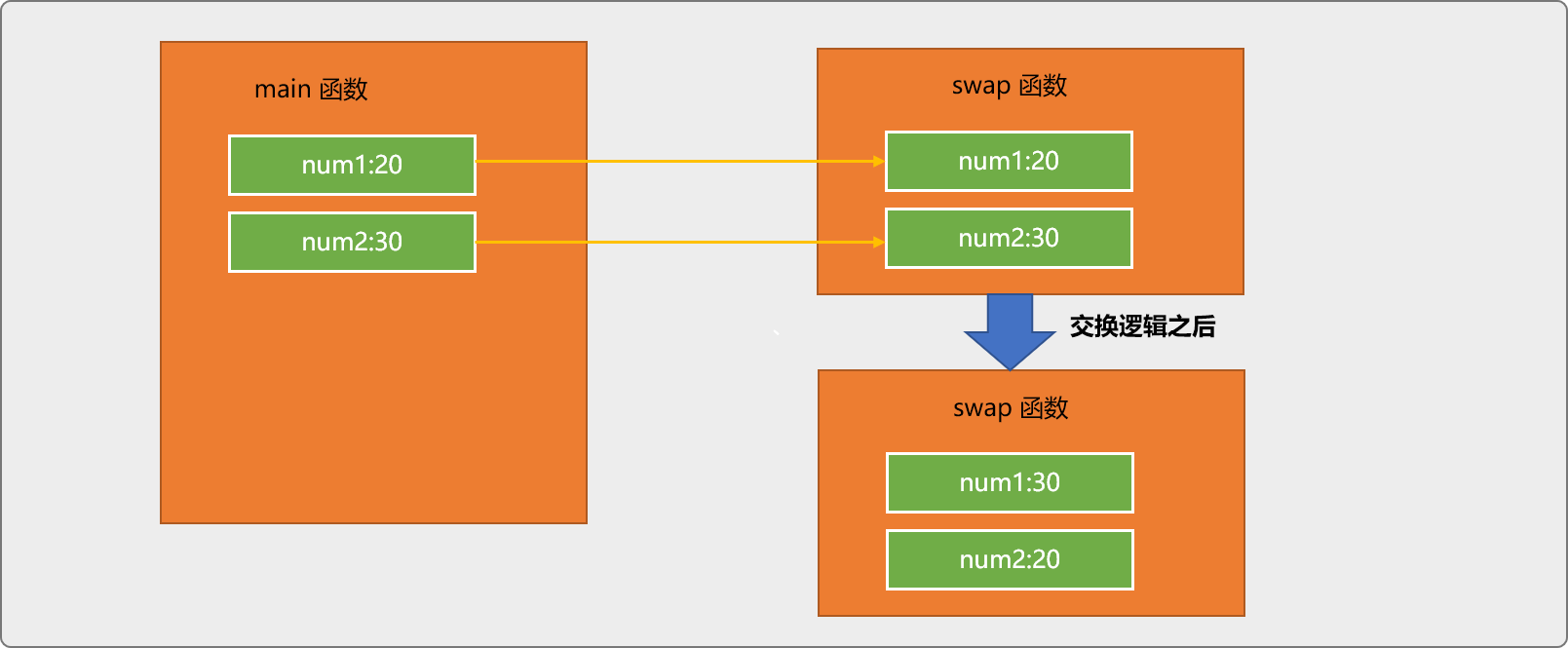``````#include <iostream>
//形参为指针类型
void swap(int* num1,int* num2){
//*num1 通过地址访问主函数中的 num1 变量
int tmp=*num1;
//交换的是主函数中变量中的值
*num1=*num2;
*num2=tmp;
}
int main(int argc, char** argv) {
int num1=20;
int num2=30;
std::cout<<"交换前："<<num1<<":"<<num2<<std::endl;
//主函数把变量的地址传递给 swap 函数
swap(&num1,&num2);
std::cout<<"交换后："<<num1<<":"<<num2<<std::endl;
return 0;
}
``````

``````交换前：20:30

``````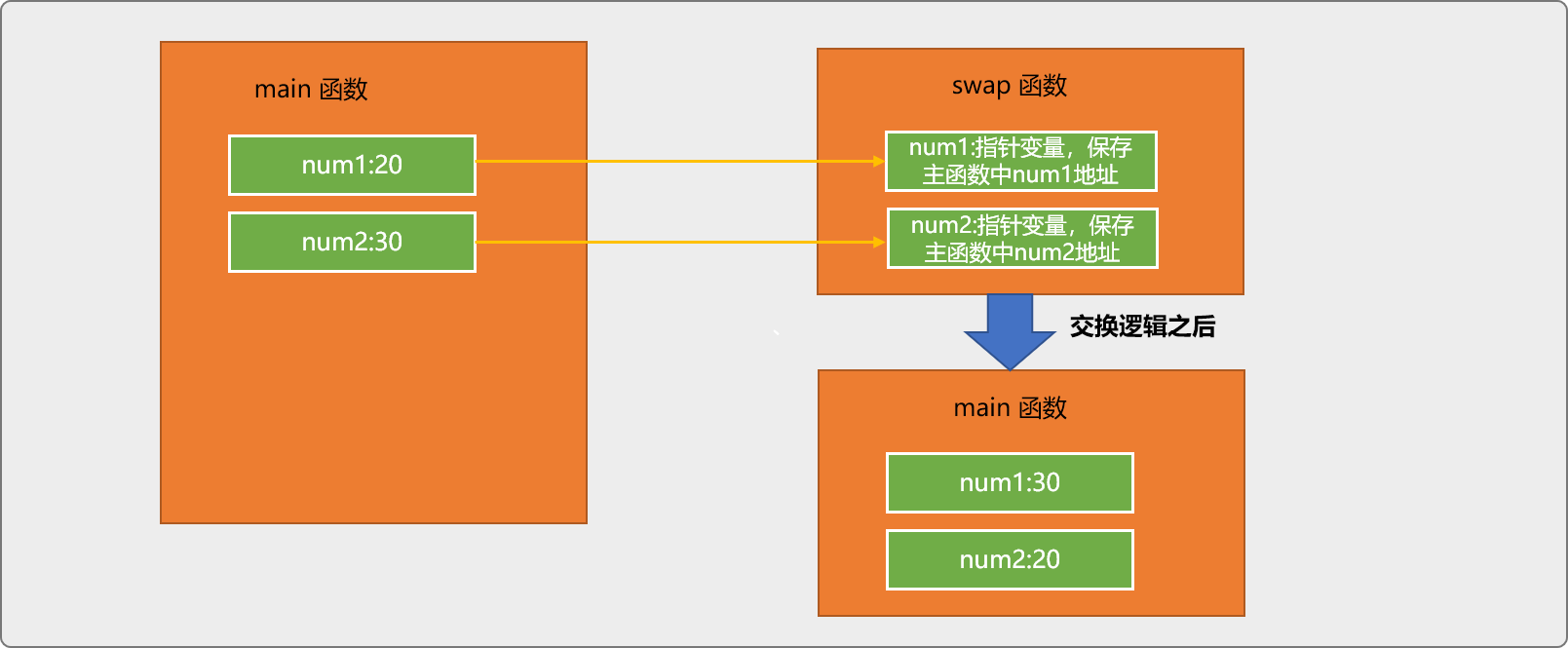### 3.2 指针潜在的风险

#### 3.2.1 初始化风险

``````int* p;
std::cout<<p<<std::endl;
std::cout<<*p<<std::endl;
``````

``````0x40ebd9
264275272
``````

``````//语法错误
int* p=0x40aed9;
``````

`0x40aed9`即使是一个有效的内存地址数据，因为类型不同，也不能把`整型数据`赋值给一个指针类型变量。

`地址`形态上是数字，也仅是形态上是，本质上不是数字类型，不具有数字语义，也不具有数字运算操作能力，不能把地址类型与数字类型混淆。

``````//正确
int* p=(int*)0x44eb99;
``````

• `0x44eb99`地址不一定是一个有效的地址。
• `0x44eb99`即使是一个有效地址，有可能此地址正被其它变量使用。如此，你便修改了其它变量的值。误打误撞，跑到了别人家里。

``````int score=89;
//本意是想使用一个空闲的空间，误打误撞引用了 score 的地址
int* p=(int*)0x70fddc;
//会修改 score 中的值
*p=56;
std::cout<<score<<std::endl;
std::cout<<*p<<std::endl;
//输出
56
56
``````

``````int num=34;
//语法错误,声明指针时的数据类型，严格规定了指针变量能引用的变量类型
double* num_p=&num;
``````

### 3.2.2 越界风险

``````int num=34;
int* num_p=&num;
std::cout<<"正常输出:"<<*num_p<<std::endl;
//指针移到了一个没有声明的区域
std::cout<<"移动指针输出:"<<*(num_p+1)<<std::endl;
``````

``````正常输出:34

``````

### 3.3 多级指针

``````//声明常规变量
int num=20;
//一级指针变量：用来保存 num 变量的地址
int* num_p=&num;
//二给指针变量，用来保存 num_p 变量的地址
int** num_p_p=&num_p;
``````

`int**`表示二级指针类型，本质还是内存地址，是另一个指针变量的内存地址。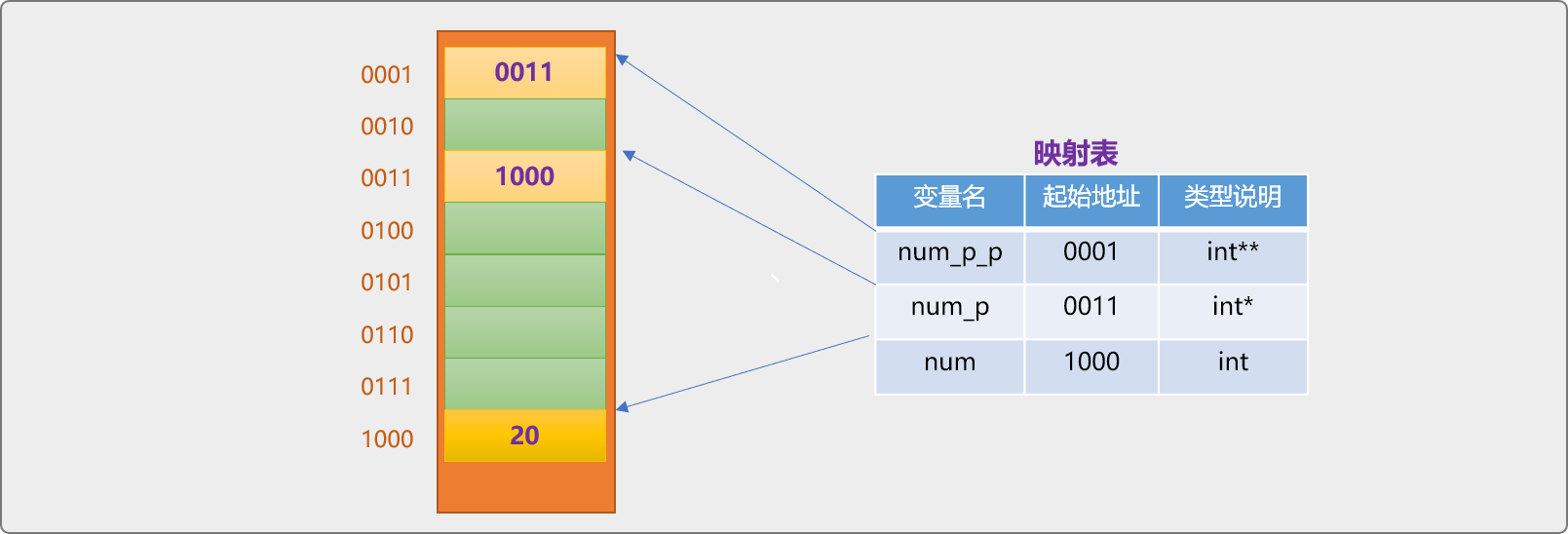``````//……
*(*num_p_p)=30;
std::cout<<"输出:"<<num<<std::endl;
``````

• `*num_p_p`获取到 `num_p`变量中的内存地址值 `1000`
• `*(*num_p_p)`利用上面返回的 `1000` 地址，找到变量 `num`位置，并返回变量 `num`的值。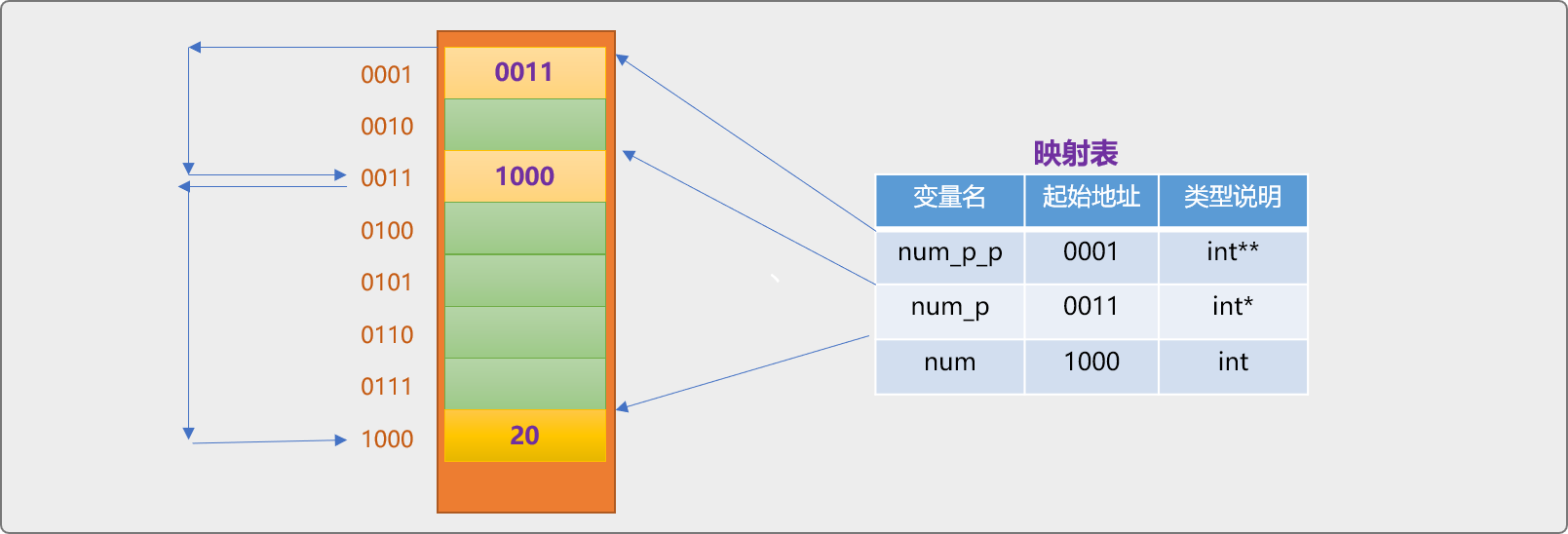``````int num=20;
int* num_p=&num;
int** num_p_p=&num_p;
int*** num_p_p_p=&num_p_p;
*(*(*num_p_p_p))=30;
std::cout<<"输出:"<<num<<std::endl;
//输出：30
``````